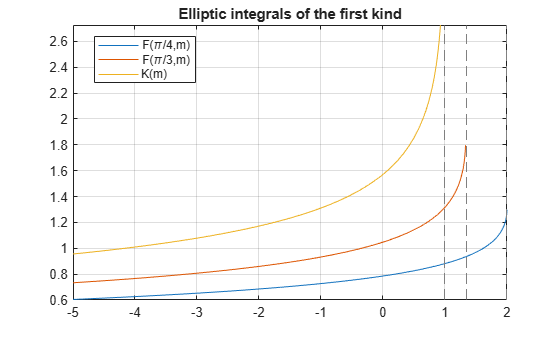# ellipticF

Incomplete elliptic integral of the first kind

## Syntax

ellipticF(phi,m)

## Description

example

ellipticF(phi,m) returns the incomplete elliptic integral of the first kind.

## Examples

### Find Incomplete Elliptic Integrals of First Kind

Compute the incomplete elliptic integrals of the first kind for these numbers. Because these numbers are not symbolic objects, you get floating-point results.

s = [ellipticF(pi/3, -10.5), ellipticF(pi/4, -pi),... ellipticF(1, -1), ellipticF(pi/2, 0)]
s = 0.6184 0.6486 0.8964 1.5708

Compute the incomplete elliptic integrals of the first kind for the same numbers converted to symbolic objects. For most symbolic (exact) numbers, ellipticF returns unresolved symbolic calls.

s = [ellipticF(sym(pi/3), -10.5), ellipticF(sym(pi/4), -pi),... ellipticF(sym(1), -1), ellipticF(pi/6, sym(0))]
s = [ ellipticF(pi/3, -21/2), ellipticF(pi/4, -pi), ellipticF(1, -1), pi/6]

Use vpa to approximate this result with floating-point numbers:

vpa(s, 10)
ans = [ 0.6184459461, 0.6485970495, 0.8963937895, 0.5235987756]

### Differentiate Incomplete Elliptic Integrals of First Kind

Differentiate this expression involving the incomplete elliptic integral of the first kind. ellipticE represents the incomplete elliptic integral of the second kind.

syms m diff(ellipticF(pi/4, m))
ans = 1/(4*(1 - m/2)^(1/2)*(m - 1)) - ellipticF(pi/4, m)/(2*m) -... ellipticE(pi/4, m)/(2*m*(m - 1))

### Plot Incomplete and Complete Elliptic Integrals

Plot the incomplete elliptic integrals ellipticF(phi,m) for phi = pi/4 and phi = pi/3. Also plot the complete elliptic integral ellipticK(m).

syms m fplot([ellipticF(pi/4, m) ellipticF(pi/3, m) ellipticK(m)]) grid on title('Elliptic integrals of the first kind') legend('F(\pi/4,m)', 'F(\pi/3,m)', 'K(m)', 'Location', 'Best')## Input Arguments

collapse all

Input, specified as a number, vector, matrix, or array, or a symbolic number, variable, array, function, or expression.

Input, specified as a number, vector, matrix, or array, or a symbolic number, variable, array, function, or expression.

collapse all

### Incomplete Elliptic Integral of the First Kind

The complete elliptic integral of the first kind is defined as follows:

$F\left(\phi |m\right)=\underset{0}{\overset{\phi }{\int }}\frac{1}{\sqrt{1-m{\mathrm{sin}}^{2}\theta }}d\theta$

Note that some definitions use the elliptical modulus k or the modular angle α instead of the parameter m. They are related as m = k2 = sin2α.

## Tips

• ellipticF returns floating-point results for numeric arguments that are not symbolic objects.

• For most symbolic (exact) numbers, ellipticF returns unresolved symbolic calls. You can approximate such results with floating-point numbers using vpa.

• At least one input argument must be a scalar or both arguments must be vectors or matrices of the same size. If one input argument is a scalar and the other one is a vector or a matrix, ellipticF expands the scalar into a vector or matrix of the same size as the other argument with all elements equal to that scalar.

• ellipticF(pi/2, m) = ellipticK(m).

 Milne-Thomson, L. M. “Elliptic Integrals.” Handbook of Mathematical Functions with Formulas, Graphs, and Mathematical Tables. (M. Abramowitz and I. A. Stegun, eds.). New York: Dover, 1972.

#### Mathematical Modeling with Symbolic Math Toolbox

Get examples and videos